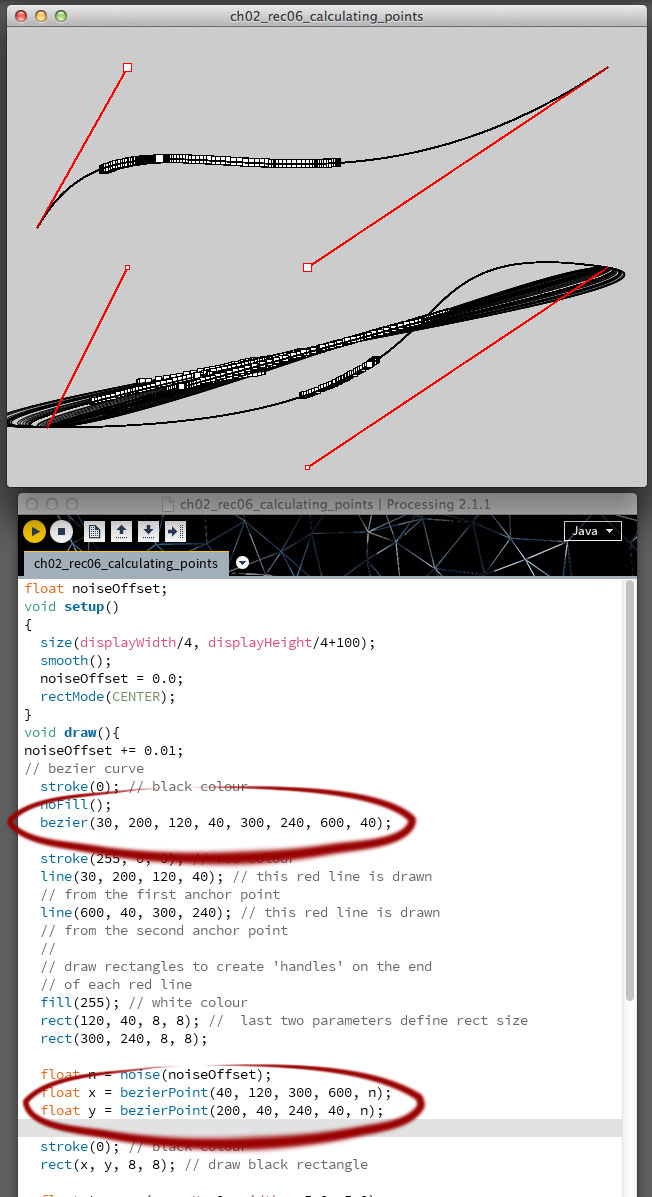# Calculating points on a curve

Making my way through the Calculating points on a curve recipe from the Processing 2 Creative programming cookbook source code on GitHub.

In this recipe I learned how to use the bezierPoint and curvePoint functions to calculate points on curves and splines. bezierPoint uses five parameters. The first four are coordinates for the anchor and control points (40, 120, 300, 600) of the bezier curve and the fifth is parameter is a number between 0 and 1. This Sketch uses noise to generate the number. curvePoint works in a similar way to bezierPoint, except it’s for Catmull-Rom splines. I also learned how to use:

• the first four parameters and the last four parameters of the bezier curve’s anchor points as the parameters for the red lines used to simulate the curve’s ‘handle’
• noise to animate the point as it’s moved along the curve
• rectMode to change the location from which the rectangle is drawn. For this example, CENTER is used to specify the first two parameters of each rect (120, 40 and 300, 240) as the shape’s centre point.